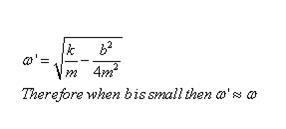Courses

# Test: Damped & Forced Oscillations

## 10 Questions MCQ Test Physics Class 11 | Test: Damped & Forced Oscillations

Description
This mock test of Test: Damped & Forced Oscillations for JEE helps you for every JEE entrance exam. This contains 10 Multiple Choice Questions for JEE Test: Damped & Forced Oscillations (mcq) to study with solutions a complete question bank. The solved questions answers in this Test: Damped & Forced Oscillations quiz give you a good mix of easy questions and tough questions. JEE students definitely take this Test: Damped & Forced Oscillations exercise for a better result in the exam. You can find other Test: Damped & Forced Oscillations extra questions, long questions & short questions for JEE on EduRev as well by searching above.
QUESTION: 1

### The phenomenon of increase in amplitude when the driving force is close to the natural frequency of the oscillator is known as

Solution:

The phenomenon of increase in amplitude when the driving force is close to the natural frequency of the oscillator is known as resonance. By the definition of resonance.

QUESTION: 2

### If ω is the natural frequency of the system and ωd is the frequency of the external force that acts on an oscillating system then at resonance

Solution:

When the natural frequency and external frequency of an object are equal the phenomenon is called resonance.

QUESTION: 3

### Under what condition angular frequency ω of the damped oscillator would be equivalent to angular velocity Ω of the undamped oscillator.

Solution:QUESTION: 4

The restoring force in a simple harmonic motion is _________ in magnitude when the particle is instantaneously at rest.

Solution:

The restoring force in a simple harmonic motion is maximum in magnitude when the particle is instantaneously at rest because in SHM object’s tendency is to return to mean position and here particle is instantaneously at rest after that instant restoring force will be max to bring particle to mean position.

QUESTION: 5

Energy is supplied to the damped oscillatory system at the same rate at which it is dissipating energy, then the amplitude of such oscillations would become constant. Such oscillations are called

Solution:

Energy is supplied to the damped oscillatory system at the same rate at which it is dissipating energy, and then the amplitude of such oscillations would become constant. Such oscillations are called maintained oscillations. By the definition of maintained oscillations.

QUESTION: 6

The amplitude of S.H.M at resonance is _______ in the ideal case of zero damping.

Solution:

In an ideal environment where there is no resistance to oscillation motion i.e. damping is zero, when we oscillate a system at its resonant frequency since there is no opposition to oscillation, the amplitude will go on increasing and reach infinity.

QUESTION: 7

What determines the natural frequency of a body?

Solution:

Natural frequency is the frequency at which a body tends to oscillate in the absence of any driving or damping force.
Free vibrations of any elastic body are called natural vibration and happen at a frequency called natural frequency. Natural vibrations are different from forced vibration which happen at frequency of applied force .

QUESTION: 8

If an external force with angular frequency Ï‰d acts on an oscillating system with natural angular frequency Ï‰, the system oscillates with angular frequency Ï‰d. The amplitude of oscillations is the greatest when:

Solution:
QUESTION: 9

Under forced oscillation, the phase of the harmonic motion of the particle and phase of driving force

Solution:

Harmonic motion is the natural motion of a body(we consider no air friction) under no force where as damped oscillation are under force hence the iscilation are different

QUESTION: 10

In the ideal case of zero damping, the amplitude of simple harmonic motion at resonance is:

Solution:

In an ideal environment where there is no resistance to oscillatory motion, that is, damping is zero, when we oscillate a system at its resonant frequency, since there is no opposition to oscillation, a very large value of amplitude will be recorded. Forced oscillation is when you apply an external oscillating force.